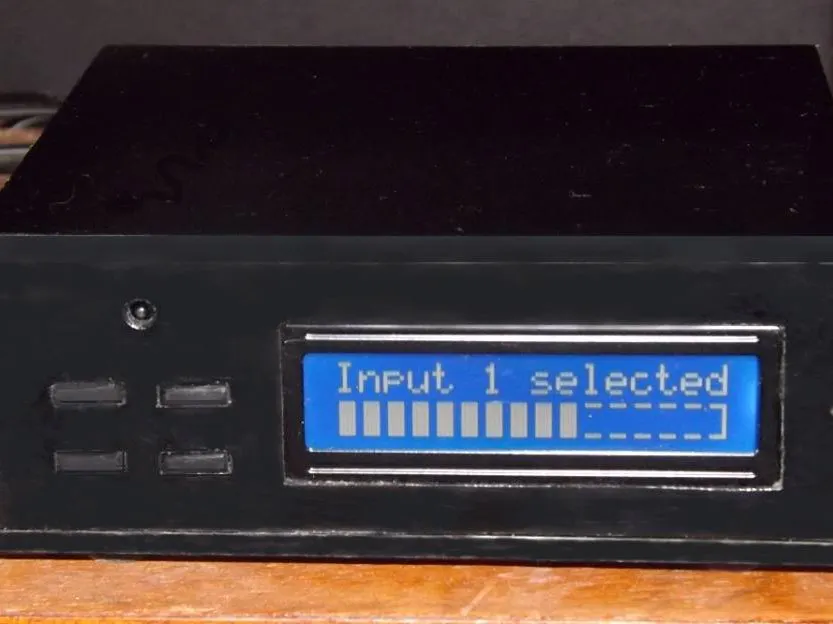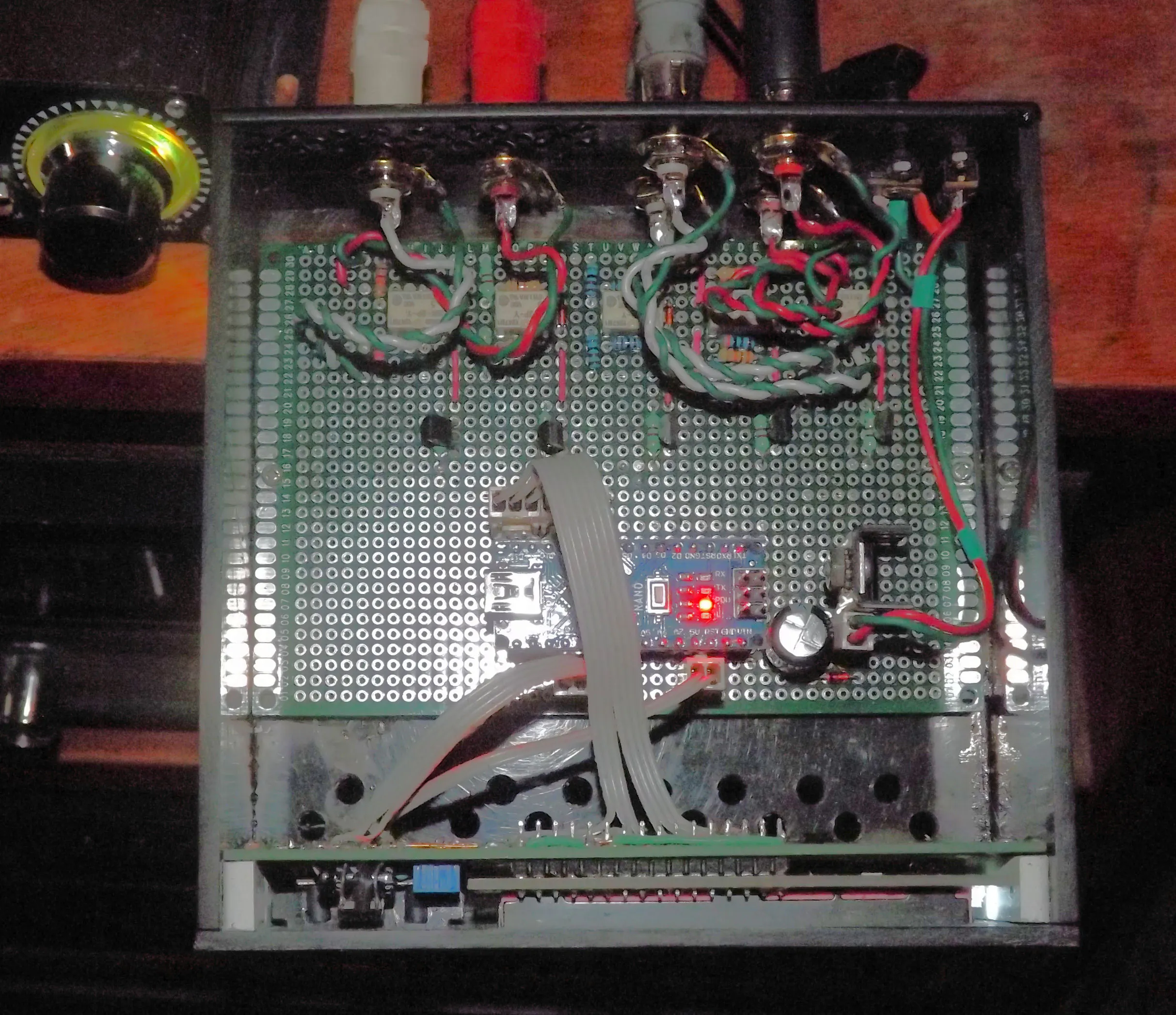# A Remote Controlled Stereo Volume Control

Using my cable TV remote, I can control the volume and select either TV or radio input to my hi-fi system with this little box.

IntermediateShowcase (no instructions)1.5 hours7,422## Things used in this project

### Hardware componentsArduino Nano R3
×1
 Molex connectors
×1

### Hand tools and fabrication machinesSoldering iron (generic)

## Schematics

### How the circuit boards are mounted in the box.## Code

### Remote Volume Control

C/C++
Copy and paste it
```#include <LiquidCrystal.h>
LiquidCrystal lcd(12, 11, 10, 9, 8, 7);
//Set custom characters for bar graph display
byte block = {0b1111,0b1111,0b1111,0b1111,0b1111,0b1111,0b1111,0b1111};
byte blank = {0b1111,0b0000,0b0000,0b0000,0b0000,0b0000,0b0000,0b1111};
byte final = {0b1111,0b0001,0b0001,0b0001,0b0001,0b0001,0b0001,0b1111};

int level=10;
int lastlevel=10;
int mflag=1;
int select;
unsigned int code=32783;
unsigned int last;

#include <IRLibDecodeBase.h>
#include <IRLib_P09_GICable.h>   //A decoder that handles only GI Cable
#include <IRLibCombo.h>
#include <IRLibRecv.h>
IRdecode myDecoder;

void setup()
{
pinMode (3, OUTPUT); //12db
pinMode (4, OUTPUT); //6db
pinMode (5, OUTPUT); //3db
pinMode (6, OUTPUT); //1.5db
pinMode (13, OUTPUT); //input select

lcd.begin(16, 2); // set up the LCD's number of columns and rows:
lcd.createChar(1, block);
lcd.createChar(2, blank);
lcd.createChar(3, final);
lcd.setCursor(0, 0);
lcd.print("Input 1 selected");

}

void loop()
{
lcd.setCursor(0, 0);
{myDecoder.decode();
code=(myDecoder.value);

if (code==65535) //Remote repeat FFFF
{code=last;}
if (code==32783) //Input 1
{lcd.print("Input 1 selected");
digitalWrite (13, LOW);
last=code;}
if (code==16391) //Input 2
{lcd.print("Input 2 selected");
digitalWrite (13, HIGH);
last=code;}
if (code==28676) //Down
{level=level-1;
last=code; lastlevel=level;}
if (code==45068) //Up
{level=level+1;
last=code; lastlevel=level;}
if (code==61448) //Mute
{last=code;
if (mflag)
{level=0; mflag=0;}
else
{level=lastlevel; mflag=1;}
}
}

lcd.setCursor(0, 0);
if (ch1 < 128)
{lcd.print("Input 1 selected");
digitalWrite (13, LOW);
}
if (ch2 < 128)
{lcd.print("Input 2 selected");
digitalWrite (13, HIGH);
}
lcd.setCursor(0, 1);

if (up < 128)
{level=level+1;}
if (dn < 128)
{level=level-1;}

//Draw the Bar Graph
if (level >=15)
{level=15;}
if (level <1)
{level=0;}
for (int i=0; i<=(level); i++)
{lcd.write (1);}
if (level < 14)
{for (int i=0; i<=(13-level); i++)
lcd.write (2);}
if (level <15)
{lcd.write (3);}

// Set the Masks for the 4 relays
select = (15 - level);

// Pin 6, 1.5db
if (select & 1)
{digitalWrite (6, HIGH);}
else
{digitalWrite (6, LOW);}
// Pin 5, 3db
if (select & 2)
{digitalWrite (5, HIGH);}
else
{digitalWrite (5, LOW);}
// Pin 4, 6db
if (select & 4)
{digitalWrite (4, HIGH);}
else
{digitalWrite (4, LOW);}
// Pin 3, 12db
if (select & 8)
{digitalWrite (3, HIGH);}
else
{digitalWrite (3, LOW);}

delay (100);